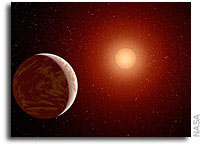# On The Eccentricity of Proxima bWe apply Monte Carlo projection to the radial velocity data set that Anglada Escude et al. (2016) use for the discovery of Proxima b. They find an upper limit to the orbital eccentricity of 0.35.

To investigate the eccentricity issue further, we calculate a suite of monovariate and bivariate densities o eccentricity. After discarding apparent artifacts at eccentricity = 0 and = 1, we find that eccentricity has a trimodal sampling distribution: three chimeras or types of orbit compatible with the RV data set. The three modes or peaks in the density of eccentricity are located at eccentricity = 0.25, 0.75, and 0.95, with relative weights 0.79, 0.10, and 0.11.

Future RV observations will clarify which of the three chimeras represents the true eccentricity of Proxima b. The most-likely estimate is eccentricity = 0.25, and our lower limit is eccentricity = 0.025. Our strategic, long-term goal is to elevate the orbital analyses of exoplanets to meet the challenges of sometimes complex probability density distributions.

Robert A. Brown
(Submitted on 15 Jan 2017)